# Simplifying Rational Expressions

### Canceling Like Factors

When we reduce a common fraction such as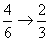we do so by noticing that there is a factor common to both the numerator and the denominator (a factor of 2 in this example), which we can divide out of both the numerator and the denominator.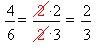We use exactly the same procedure to reduce rational expressions.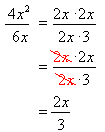#### Polynomial / Monomial

Each term in the numerator must have a factor that cancels a common factor in the denominator.but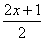cannot be reduced because the 2 is not a common factor of the entire numerator.

WARNING  You can only cancel a factor of the entire numerator with a factor of the entire denominator

 However, as an alternative, a fraction with more than one term in the numerator can be split up into separate fractions with each term over the same denominator; then each separate fraction can bereduced if possible:· Think of this as the reverse of adding fractions over a common denominator. Sometimes this is a useful thing to do, depending on the circumstances.You end up with simpler fractions, but the price you pay is that you have more fractions than you started with.
• Polynomials must be factored first. You can’t cancel factors unless you can see the factors:

Example: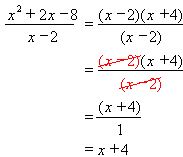• Notice how canceling the (x– 2) from the denominator left behind a factor of 1

### Multiplication and Division

Same rules as for rational numbers!

#### Multiplication

• Both the numerators and the denominators multiply together
• Common factors may be cancelled before multiplying

Example:

 Given Equation: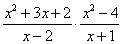First factor all the expressions: (I also put the denominators in parentheses because then it is easier t  see them as distinct factors)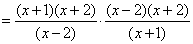Now cancel common factors—any factor on the top can cancel with any factor on the bottom: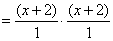Now just multiply what’s left. You usually do not have to multiply outthe factors, just leave them as shown.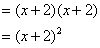#### Division

• Multiply by the reciprocal of the divisor
• Invert the second fraction, then proceed with multiplication as above
• Do not attempt to cancel factors before it is written as a multiplication

Same procedure as for rational numbers!

• Only the numerators can be addedtogether, and only when all the denominators are the same

#### Finding the LCD

• The LCD is built up of all the factors of the individual denominators, each factor included the most number of times it appears in an individual denominator.
• The product of all the denominators is always a commondenominator, but not necessarily the LCD (the final answer may have to be reduced).

Example:

 Given equation: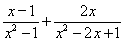Factor both denominators: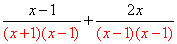Assemble the LCD: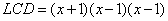Note that the LCD contains bothdenominators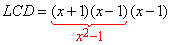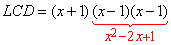Build up the fractions so that they both have the LCD for a denominator: (keep both denominators in factored form to make it easier to see what factors they need to look like the LCD)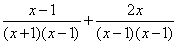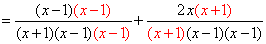Now that they are over the same denominator, you can add the numerators: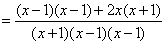And simplify: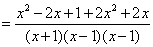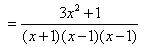Confused by Simplifying Rational Expressions in math? Get professional assignment help to clarify your understanding and excel!

If you need someone to do your math homework , you can turn to CWassignments.com.  Our experts can help you with all kinds of STEM assignments including ‘do my programming homework‘ help.